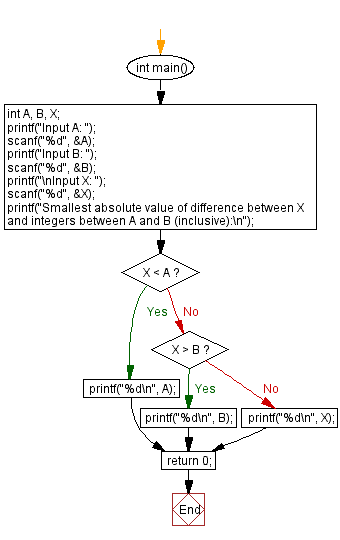﻿ C - The smallest absolute difference between X and 2 integers

# C Exercises: The smallest absolute difference between X and 2 integers

## C Basic-II: Exercise-7 with Solution

Write a C program that accepts three integers A, B, X. Out of the integers between A and B (inclusive) find the smallest absolute value of difference from X.

Sample Date:

Input A, B: 7, 11
C: 20
Smallest absolute value of difference between X and integers between A and B (inclusive): 11
Input A, B: 1, 5
C: 4
Smallest absolute value of difference between X and integers between A and B (inclusive): 4

C Code:

``````#include <stdio.h>

int main()
{
int A, B, X;
printf("Input A: ");
scanf("%d", &A);
printf("Input B: ");
scanf("%d", &B);
printf("\nInput X: ");
scanf("%d", &X);

printf("Smallest absolute value of difference between X and integers between A and B (inclusive):\n");

if (X < A){
printf("%d\n", A);
}
else if (X > B){
printf("%d\n", B);
}
else {
printf("%d\n", X);
}

return 0;
}
``````

Sample Output:

```Input A: 7
Input B: 11

Input X: 20
Smallest absolute value of difference between X and integers between A and B (in
clusive):
11

```

Flowchart:C Programming Code Editor:

Previous C Programming Exercise: Length of longest ascending contiguous subsequence.

What is the difficulty level of this exercise?

Test your Programming skills with w3resource's quiz.

﻿

```MY_TYPE a = { .flag = true, .value = 123, .stuff = 0.456 };